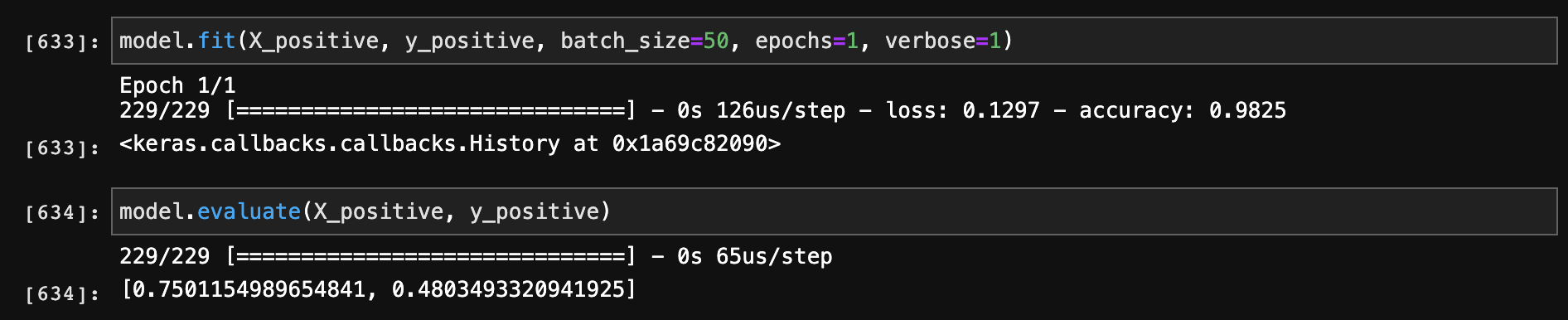#### Keras模型针对同一数据集做fit和evaluation，accuracy值却不相同

统计/机器学习 人工神经网络 TensorFlow    浏览次数：1265        分享

1

inputs = Input(shape=(X_train.shape,))
nb_x_1 = BatchNormalization()(inputs)
dense_x_1 = Dense(64, activation='relu')(nb_x_1)
nb_x_2 = BatchNormalization()(dense_x_1)
dense_x_2 = Dense(64, activation='relu')(nb_x_2)
predictions = Dense(1, activation='sigmoid')(dense_x_2)

model = Model(inputs=inputs, outputs=predictions)
model.compile(optimizer=optmzr,
loss="binary_crossentropy",
metrics=['accuracy'])macavity   2020-08-04 22:02

##### 1个回答
1

BatchNorm() 在evaluation下会被关掉

SofaSofa数据科学社区DS面试题库 DS面经anlijuncn   2020-08-11 16:07相关主题